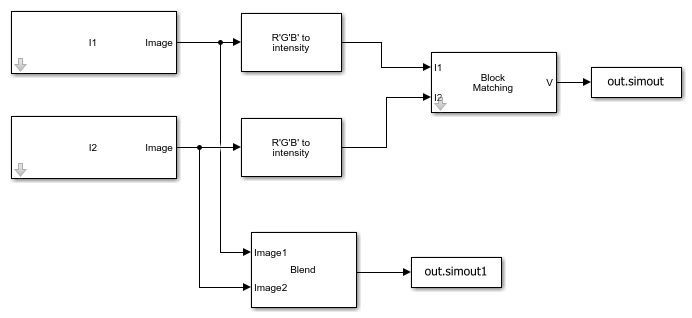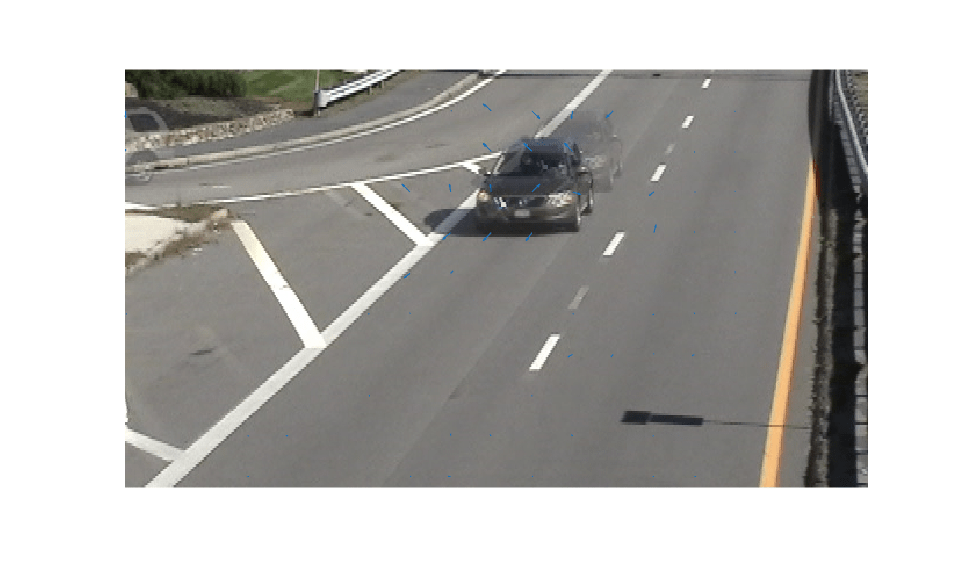# Estimate Motion between Two Images

This example shows how to use the `Block` `Matching` block to estimate motion between two images.

Read image frames for which motion has to be estimated.

```I1 = imread('car_frame1.png'); I2 = imread('car_frame2.png'); ```

### Example Model

Open the model by calling the `open` function in MATLAB command prompt. Specify the name of the Simulink file to open.

```open_system('ex_blockmatching.slx'); ```Load the images into the model workspace by using the `Image From Workspace` block. To directly read images from a file location, use the `Image From File` block instead. The model estimates motion between two RGB images of a moving car that are captured at different time intervals. The model uses the three step block matching algorithm for motion estimation. The cost function for matching the non-overlapping macro blocks is set to mean square error (MSE). The size of the macro blocks is set to 35-by-35 and maximum displacement (in horizontal and vertical direction) allowed for the matching blocks is set to 7 pixels. The velocity output from the `Block` `Matching` block consists of both the horizontal and vertical components of the motion vector in complex form.

You can use the `Compositing` block to overlay both the images.

### Run Model

Simulate the model and save the model output to MATLAB workspace. The model outputs the motion vector and the overlayed image.

```out = sim('ex_blockmatching.slx'); ```

### Display Results

Read the output motion vector and the overlayed image.

```vx = real(out.simout.Data); vy = imag(out.simout.Data); imageOverlay = out.simout1.Data; ```

Specify the points on the image plane relative to the size of the macro blocks.

```x = 1:35:size(imageOverlay,1); y = 1:35:size(imageOverlay,2); ```

Display the overlayed image and plot the horizontal and vertical components of the motion vector by using the `quiver` function.

```figure,imshow(imageOverlay); hold on quiver(y',x,vx,vy,0); ```